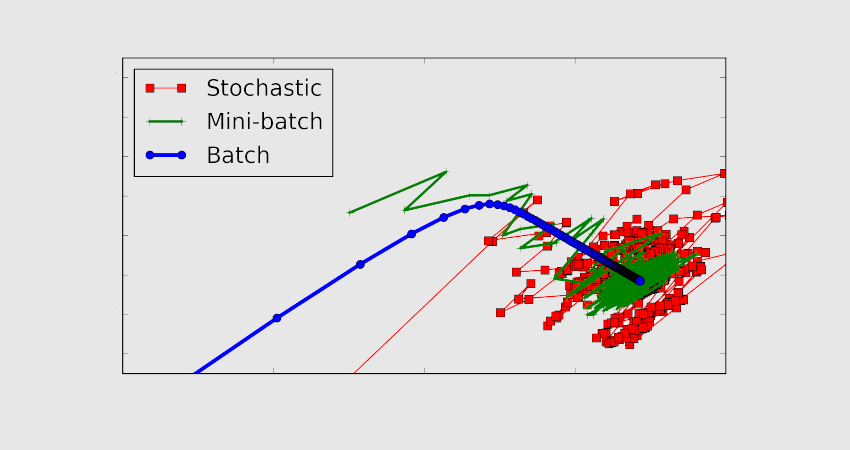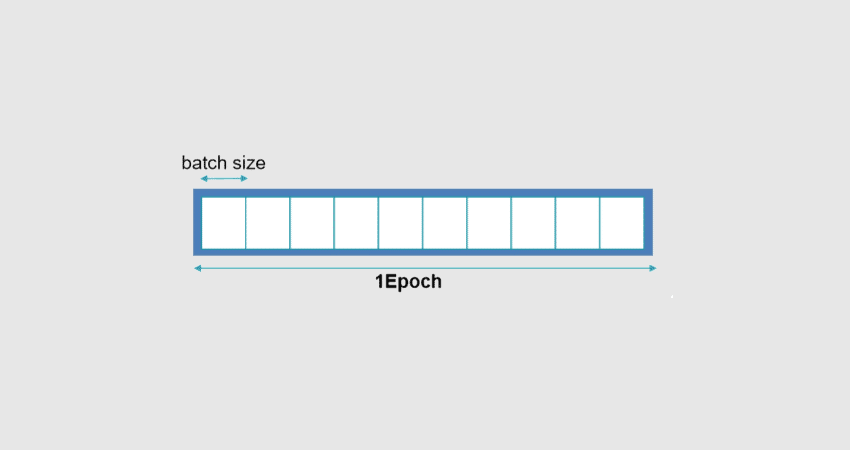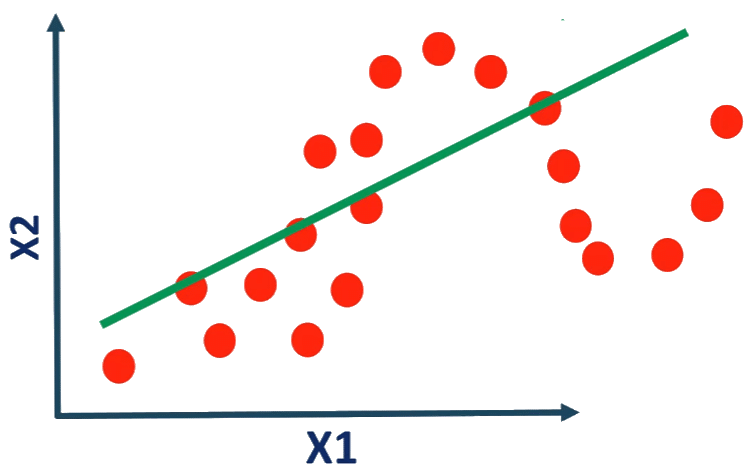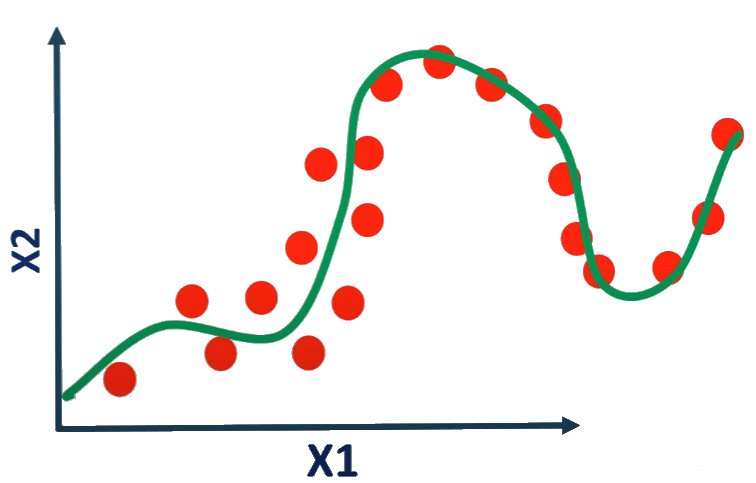#### We have the answers to your questions! - Don't miss our next open house about the data universe!# Epoch : An essential notion in real-time programming## An epoch in Machine Learning refers to one complete pass of the training data set through the algorithm. Learn all you need to know about this essential concept of machine learning.In the field of artificial intelligence, Machine Learning involves allowing a model to learn and train from data using an algorithm. This method is inspired by the way the human brain learns, and is based on artificial neural networks.

However, while a human needs several years to learn, through techniques such as parallel training, a model can train for the equivalent of several decades in just a few hours.

Furthermore, each time the training dataset passes through the algorithm, it is said to have completed an epoch. This is a hyperparameter, determining the training process of the Machine Learning model.

### What is an Epoch?

One complete pass of the training dataset is considered an “epoch” in the field of Machine Learning. It reflects the number of passes of the algorithm during the training phase.

An epoch can be defined as the number of passes of a training dataset through an algorithm. One pass is equivalent to a round trip. The number of epochs can reach several thousand, as the procedure is repeated indefinitely until the model’s error rate is reduced to an acceptable level.

An epoch is made up of an aggregation of “batches” of data and iterations. Data sets are generally broken down into batches, particularly when the volume of data is massive.### What is an iteration?

In the Machine Learning field, an iteration indicates the number of times that the parameters of an algorithm are modified. The specific implications depend on the context.

Generally, a training iteration of a neural network includes batch processing or processing of the dataset in batches, calculation of the cost function, modification and backpropagation of all weight factors.

Iteration and epoch are often wrongly confused. In reality, an iteration involves the processing of a batch while an epoch refers to the processing of all the data in the dataset.

For example, if an iteration processes 10 images of a set of 1000 images with a batch size of 10, it will take 100 iterations to complete one epoch.

### What is a batch?

Training data is broken down into several small “batches.” The goal is to avoid problems related to lack of storage space.

Batches can be easily used to feed the Machine Learning model in order to train it. This process of breaking down the dataset is called “batch processing“.

One epoch can be made up of one batch or more. The number of training samples used in one iteration is the “batch size”. There are three possibilities :

• In “batch mode,” the values of iteration and epoch are equal since the batch size is equal to the complete dataset. Therefore, one iteration is equivalent to one epoch.
• In “mini-batch mode“, the size of the complete dataset is smaller than the batch size. Therefore, a single batch is larger than the training dataset.

Finally, in “stochastic mode“, the batch size is unique. Therefore, the gradient and parameters of the neural network are changed with each sample.### What is the stochastic gradient descent algorithm?

The Stochastic Gradient Descent (SGD) algorithm is an optimization algorithm. It is used by neural networks in the field of Deep Learning to train Machine Learning algorithms.

The role of this algorithm is to identify a set of internal model parameters that exceed other performance measures such as the mean squared error or log loss.

Optimization can be described as a search process involving learning. The optimization algorithm is called gradient descent, and it is based on the calculation of an error gradient or error slope that needs to be descended in the direction of the minimum error level.

This algorithm allows the search process to be run multiple times. The aim is to improve the model’s parameters at each step. Therefore, it is an iterative algorithm.

At each step, predictions are made using specific samples using the set of internal parameters. The predictions are then compared to the expected results to calculate the error rate. The internal parameters are then updated.

### JUMPSTART YOUR CAREER IN A DATA SCIENCE

Are you interested in a career change into Big Data, but don’t know where to start? Then you should take a look at our Data Science training course### JUMPSTART YOUR CAREER IN A DATA SCIENCE

Are you interested in a career change into Big Data, but don’t know where to start?

Then you should take a look at our Data Science training courseDifferent algorithms use different update procedures. In the case of artificial neural networks, the algorithm uses the backpropagation method.

### Batch size vs epoch: what's the difference?

The batch size is the number of samples processed before the model changes. The number of epochs is the number of complete iterations of the training dataset.

A batch must have a minimum size of one and a maximum size that is less than or equal to the number of samples in the training dataset.

For the number of epochs, it is possible to choose an integer value between one and infinity. The processing can be run indefinitely and even be stopped by criteria other than a predetermined number of epochs. For example, the criterion may be the model’s error rate.

The batch size and number of epochs are hyperparameters of the learning algorithm and must be indicated to the algorithm. These parameters must be configured by testing many values to determine which is optimal.### How to choose the number of epochs?

After each iteration of the neural network, the weights are modified. The curve evolves from underfitting to overfitting, passing through the ideal fit. The number of epochs is a hyperparameter that must be decided before training begins.

A larger number of epochs does not necessarily lead to better results. Generally, a number of 11 epochs is ideal for training on most datasets.

Learning optimization is based on the iterative process of gradient descent. This is why a single epoch is not enough to optimally modify the weights. On the contrary, an extra epoch can cause the model to overfit…

### Why is epoch essential in Machine Learning?

The epoch is one of the crucial concepts in Machine Learning. It helps to identify the model that represents the data as faithfully as possible.

The neural network must be trained based on the number of epochs and batch size indicated to the algorithm. This hyperparameter therefore determines the entire course of the process.

In any case, there is no secret recipe or magic formula for defining the ideal value of each parameter. Data analysts have no choice but to test a wide variety of values before choosing the one that is best suited to solving the specific problem.

One method for determining the appropriate number of epochs is to monitor learning performance by comparing this number with the model’s error rate. The learning curve is very useful for checking whether a model is overfitting, underfitting, or adequately trained.### How to follow a Machine Learning course?

In summary, the epoch is a term used to describe the frequency at which training data passes through the algorithm. It is one of the essential concepts of Machine Learning.

To acquire expertise in this field, you can choose DataScientest. Our Data Scientist, Data Analyst, and Machine Learning Engineer courses all include a module dedicated to Machine Learning.

You will discover supervised and unsupervised learning, classification techniques, regression, clustering with scikit-learn, or even Text Mining, time series, and dimensionality reduction.

Through the other modules of these curricula, you can acquire all the skills required to become a professional in Data Science. You can pursue careers as a Data Analyst, Data Scientist, or Machine Learning Engineer depending on the chosen path.

All our courses are fully conducted online via the internet and can be completed as Continuing Education or in a BootCamp. Our innovative Blended Learning approach combines distance learning on a coached platform and Masterclass.

At the end of the course, learners receive a certificate from Mines ParisTech PSL Executive Education and validate block 3 of the RNCP 36129 “AI Project Manager” certification recognized by the French state. You can also take exams to obtain Microsoft Certified Power Platform Fundamentals or AWS Cloud Practitioner certifications.

You know everything about the epoch in the field of Machine Learning. For more information on the same subject, discover our complete file on TensorFlow, a machine learning framework.

### You are not available?

Leave us your e-mail, so that we can send you your new articles when they are published!